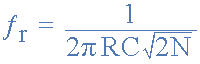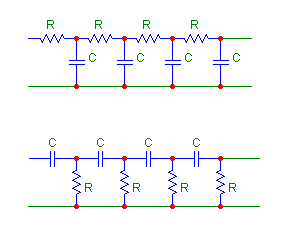# rc phase shift oscillator

Status
Not open for further replies.

#### electroboy

##### Member level 5
the frequency of oscillation of a rc phaseshift oscillator iswhere N is the number of rc stages....
please tell me how we can arrive at this expression ..... i can't find out a proper derivation...thanks in advance..

Î doubt, that it's correct. Which oscillator topology is meant exactly?

•kavikannan

### kavikannan

Points: 2

The expression (for a high-pass RC oscillator) is correct for N=3. If you increase the number of RC stages, the oscillation frequency increases. So the general expression is obviously wrong.

•electroboy

### electroboy

Points: 2
thank you .. can u please tell me how to select R and C accordingly for 4 stage rc to get 180 degree phase shift ,say for 1KHz frequency??

and also.. in 3 stage .. can i assume C and find R using the above eqn.. or should there be any other relation between r and c for 180 degree phase shift ?

As said, the frequency expression for the 3 stage RC-oscillator in the link is correct, you'll also find it at Wikipedia (with an exact derivation). But the network equations for the coupled RC circuit are rather long winded. Thus I prefer a simulator (e.g. LTSpice) to calculate the transmission function and oscillation frequency.

•electroboy

### electroboy

Points: 2
thank you .. can u please tell me how to select R and C accordingly for 4 stage rc to get 180 degree phase shift ,say for 1KHz frequency??

and also.. in 3 stage .. can i assume C and find R using the above eqn.. or should there be any other relation between r and c for 180 degree phase shift ?

Have a look at this link:

An expression for the 4 stage network is given there:

"Calculate R to complement C at oscillation frequency. There is no trivial way to estimate the oscillation frequency better than within a factor of two or so short of using the derived equations. A 3-section RC uses f = 1 / (2 * pi * SQRT(6) * R * C), but we have a four section RC, so we use the following: *

f = 1 / (2 * pi * SQRT(10 / 7) * R * C) (for a 4-section RC)
R = 1 / (2 * pi * SQRT(10 / 7) * f * C)"

You can also find a description of a method for solving the network here:

http://www.crbond.com/papers/ent23.pdf

•electroboy

### electroboy

Points: 2
Hi Electrician, the given formula for the 4-element phase shift oscillator is not correct. The factor in the denominator D must be: SQRT(7/10)
In case somebody is interested in the derivation - here it is:

The transfer function H(s) for 4 equal RC sections (T=RC) in series can be calculated (by hand or using a symbolic analyzer ) to be:

H(s)=1/D(s) with D(s)=1 + 10*(sT) + 15*(sT)^2 + 7*(sT)^3 + (sT)^4.

At resonant frequency H(jw) must be negative and real (phase shift -180deg) - thus IMG(D(jw))=0

After replacing s=jw we get a simple equation:

10wT-7(wT)^3=0

w=(1/T)*SQRT(10/7)=1/(T*SQRT(7/10))

•electroboy

### electroboy

Points: 2
There are two possible ways to arrange a 4 stage phase shift network using equal Rs and Cs; see the image. You have obtained a result for one of them. Repeat your calculations for the other topology and compare the results. Then look again at the circuit in the web page:

and tell me if you still think there is an error there.•electroboy

### electroboy

Points: 2
Results from a simulation are:

For the first circuit (RCRCRCRC):
F = 1 / (2 * pi * SQRT(7 / 10) * R * C)

For the second circuit (CRCRCRCR):
F = 1 / (2 * pi * SQRT(10 / 7) * R * C)

•electroboy

### electroboy

Points: 2
What simulation software did you use to obtain those results?

•electroboy

### electroboy

Points: 2
I used the free version of SIMetrix SIMPLIS to look at phase shift vs frequency for the two circuits you showed in post 9, with R=10K and C=100nF.

One of the formulas predicted F=190.23Hz, while the other predicted F=133.16. In the simulation, those were the frequencies where phase shift=180 degrees for the two circuits. So - good agreement between theory and simulation.

If you're looking for good free simulation software, I recommend SIMetrix SIMPLIS.

Free simulation software I've tried:
• CircuitMaker "student edition":
Very easy to use, with a nice user interface, but has serious bugs - sometimes gives wildly wrong answers.
• LTSpice:
Very good but difficult to use - has a user interface from hell IMHO.
• SIMetrix SIMPLIS:
Good and easy to use - best of both worlds.

•electroboy

### electroboy

Points: 2
There are two possible ways to arrange a 4 stage phase shift network using equal Rs and Cs; see the image. You have obtained a result for one of them. Repeat your calculations for the other topology and compare the results. Then look again at the circuit in the web page:
and tell me if you still think there is an error there.

OK, I see - and I agree that the formula cited by you was for a high-pass phase shift network.
On the other hand, I must confess, thinking of a phase shift oscillator only the low-pass version comes into my mind immediately (because of its advantages if compared with the high-pass version).
Therefore, I have derived the formula for this kind of phase shift network only. But, of course, the same principle can be applied for the high-pass section.
By the way: In post#8 once I have used the term "resonant frequency". This obviously is not correct. It is simply the "oscillation frequency".
LvW

•electroboy and goldsmith

Points: 2